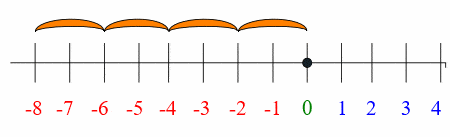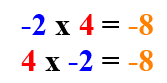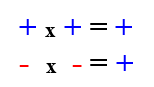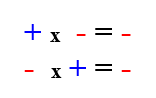# Multiplying Positive and Negative Numbers: 3 Simple Rules

There are less rules when multiplying positive and negative numbers than in adding and subtracting positive and negative numbers. There are only three rules to remember:

## Rule 1: A positive number times a positive number equals a positive number.

This is the multiplication you have been doing all along, positive numbers times positive numbers equal positive numbers.

For example, 5 x 3 = 15. 5 is a positive number, 3 is a positive number and multiplying equals a positive number: 15.

The answer is 5 x 3 = 15.

## Rule 2: A negative number times a positive number equals a negative number.

When you multiply a negative number to a positive number, your answer is a negative number. It doesn’t matter which order the positive and negative numbers are in that you are multiplying, the answer is always a negative number.

For example: -2 x 4, which in essence is the same as -2 + (-2) + (-2) + (-2)The answer is -2 x 4 = -8.

And as we said, if it’s the other way around 4 x -2, the answer is still the same: -8.The answer is 4 x -2 = -8.

## Rule 3: A negative number times a negative number, equals a positive number.

Two negatives make a positive, so a negative number times a negative number makes a positive number. If you look at it on the number line, walking backwards while facing in the negative direction, you move in the positive direction.

For example. -2 x -4 are both negative, so we know the answer is going to be positive.

The answer is -2 x -4 = 8.

Here’s the overall rule to remember when multiplying positive and negative numbers:

Two like signs make a positive sign:Two unlike signs make a negative sign:If you’re still confused over why a negative number times a negative number makes a positive number, Diana Brown at the Department of Mathematics, the University of Georgia, explains it in many different ways in this article.

Scott at About.com also has put together a handy video on how to create a cheat sheet for multiplying negative and positive numbers (scroll down the page and you’ll find the video).